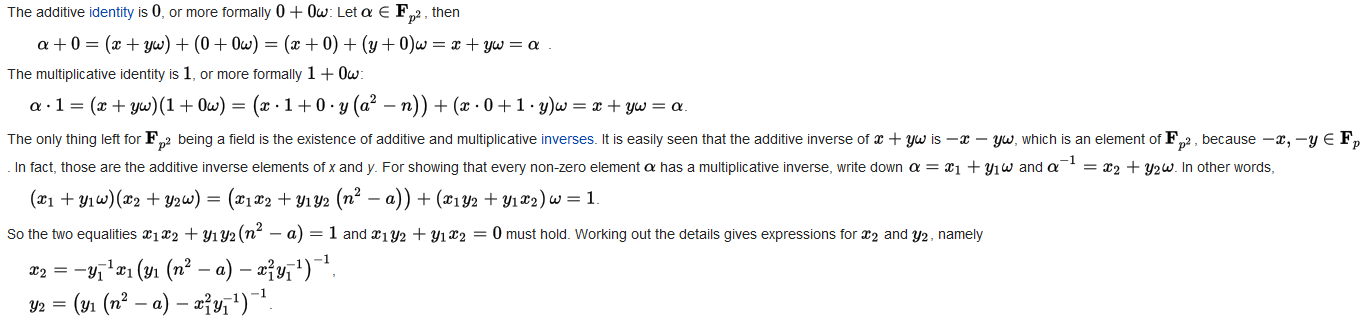# Paper

• 定理1：对于x2n(modp)$x^2\equiv n\pmod p$，总共有p12$\frac{p-1}{2}$个的n$n$能使该方程有解（将n=0$n=0$情况除去，由于该情况显然有x=0$x=0$）。
• 证明：我们只用考虑所有x2$x^2$。如果存在不同的两个数u$u$v$v$，它们的平方在模p$p$意义下同余，那么显然有p|(u2v2)$p|(u^2-v^2)$。由平方差公式p|(u+v)(uv)$p|(u+v)(u-v)$。显然p$p$不可能整除uv$u-v$，因此p$p$整除u+v$u+v$，因此u+v0(modp)$u+v\equiv0\pmod p$。这个结论反过来也是成立的，因此共有p12$\frac{p-1}{2}$种互不相同的平方，显然对应了所有有解的n$n$，而且同一个n$n$还一定存在两个互为相反数的解。

(ap)=1,1,0,apapa0(modp)

• 定理2：(ap)ap12(modp)$\left(\frac{a}{p}\right)\equiv a^{\frac{p-1}{2}}\pmod p$
• 证明：
• a$a$在模p$p$意义下是二次剩余时，令x2a(modp)$x^2\equiv a\pmod p$，那么就有xp11(modp)$x^{p-1}\equiv1\pmod p$，由费马小定理，显然x$x$存在。
• a$a$在模p$p$意义下不是二次剩余时，依旧令x2a(modp)$x^2\equiv a\pmod p$，那么就有xp11(modp)$x^{p-1}\equiv-1\pmod p$，由费马小定理，显然x$x$不存在。
• a0(modp)$a\equiv0\pmod p$时显然满足。x(a+ω)p+12(modp)$x\equiv (a+\omega)^\frac{p+1}{2}\pmod p$

• 定理3：ωpω(modp)$\omega^p\equiv -\omega\pmod p$
• 证明：ωpω×ωp1ω×(ω2)p12ω×(a2n)p12ω(modp)$\omega^p\equiv \omega\times\omega^{p-1}\equiv\omega\times(\omega^2)^{\frac{p-1}{2}}\equiv\omega\times\left(a^2-n\right)^{\frac{p-1}{2}}\equiv-\omega\pmod p$
• 定理4：(a+b)nan+bn(modn)(nP)$(a+b)^n\equiv a^n+b^n\pmod n(n\in P)$
• 证明：使用二项式定理我们能得到
(a+b)ni=0nCinaibni(modn)
由于n$n$是质数，因此当i$i$不等于0$0$且不等于n$n$的时候，组合数阶乘公式中的n$n$是没有办法被消掉的，就会被模成0$0$，因此这些项都是对答案没有贡献的。而i=0$i=0$i=n$i=n$时，我们就分别可以得到an$a^n$bn$b^n$，定理得证。

x2(a+ω)p+1(a+ω)p(a+ω)(ap+ωp)(a+ω)(aω)(a+ω)aap11(modp)a2ω2a2(a2n)n(modp)

# Problems

z$z$给我们讲了CodeChef上面一道丧心病狂的BSGS$BSGS$（离散对数）和Cipolla$Cipolla$（二次剩余）连用的题目FN